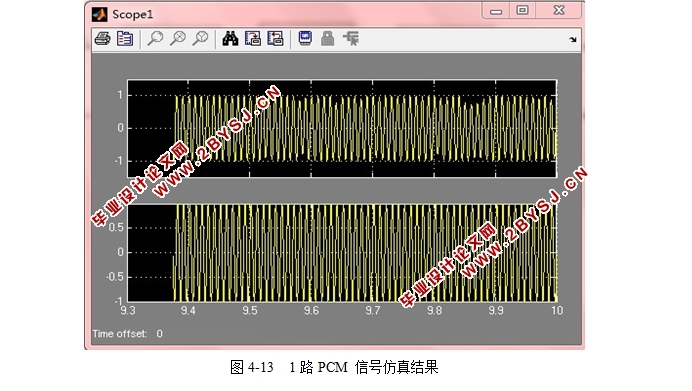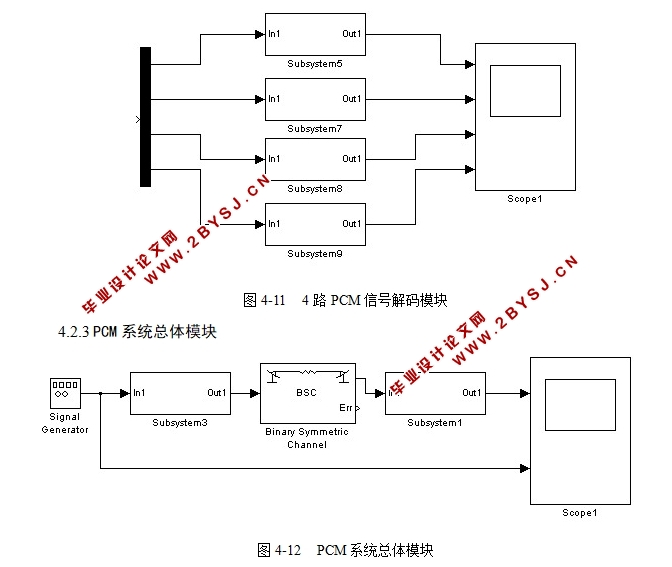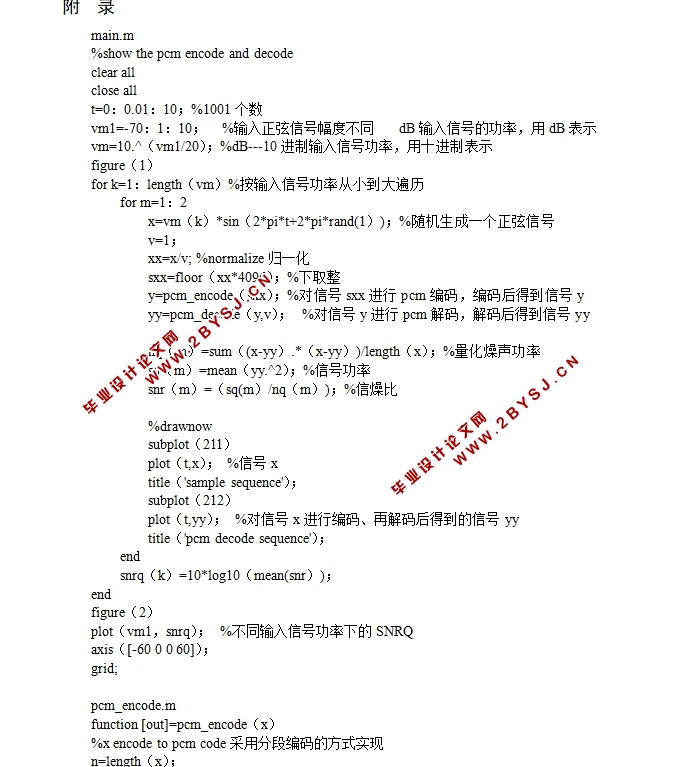# 脉冲编码调制通信系统仿真设计(MATLAB)(附程序)

1.无需注册登录,购买下载后即可获取该完整的资料.
2.购买后本站提供下载链接或联系客服发送资料.

Simulation Design of Pulse Coded Modulation Communication System
Abstract This paper describes the theoretical basis and implementation method of pulse coded modulation technology.The spectrum of sampled signal is simulated by MATLAB. Sampling conditions satisfying different conditions are analyzed and Nyquist sampling theorem is verified. The non-uniform quantization compression technology and coding in PCM modulation system are simulated and calculated by calculating signal-to-noise ratio at different quantization levels. The simulation model of pulse coded modulation is established by using the visualization tool provided by MATLAB. The design of each module is described in detail, and the problems needing attention in simulation modeling are pointed out. Under the given simulation conditions, the simulation program is run and the expected simulation results are obtained. Through the simulation and calculation of sampling, quantization and coding of PCM modulation system with MATLAB language, the analysis of PCM modulation system becomes simple. The application of MATLAB language in communication simulation will be more and more extensive.
Key words Sampling theorem； Nonuniform quantization； MATLAB； simulation1 前言    1
2 PCM编码系统介绍    1
2.1信号源子系统的组成    1
2.2 PCM编码器模块    5
3运用MATLAB仿真分析PCM调制系统    6
3.1均匀量化的仿真    6
3.2非均匀量化的仿真    8
4系统仿真    10
4.1 总体设计思想    10
4.2 仿真波形    11
5设计过程中需要解决的问题    17